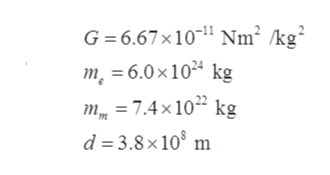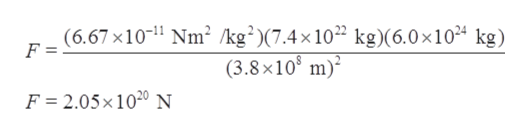# Find the force of gravity between Earth (mass=6.0 x 10^24kg) and the Moon (mass=7.4 x 10^22kg). The average Earth-Moon distance is 3.8 x 10^8m.Express your answer to two singificant figures and include the appropriate units.

Question
41 views

Find the force of gravity between Earth (mass=6.0 x 10^24kg) and the Moon (mass=7.4 x 10^22kg). The average Earth-Moon distance is 3.8 x 10^8m.

Express your answer to two singificant figures and include the appropriate units.

check_circle

Step 1

Given,help_outlineImage TranscriptioncloseG= 6.67x101 Nm2 /kg2 m 6.0x104 kg = 7.4x 10 kg d 3.8x 10 m fullscreen
Step 2

Force of gravity (F) with mass of earth as me and mass of sun as ms and d is the average distance from earth to moon. It can be evaluated as:

Step 3

Now, plugging the given and d...help_outlineImage Transcriptionclose(6.67 x1011 Nm2 /kg2 )(7.4x1022 kg)(6.0 x1024 kg) (3.8x108 m) F = F 2.05x 1020N fullscreen

### Want to see the full answer?

See Solution

#### Want to see this answer and more?

Solutions are written by subject experts who are available 24/7. Questions are typically answered within 1 hour.*

See Solution
*Response times may vary by subject and question.
Tagged in
SciencePhysics

### Gravitation